Takes an image and applys a convolution operation to it, using a user-supplied or built-in kernel. Edges are calculated by limiting the size of the kernel to only that overlapping the actual image (renormalizing the kernel for the edges).

render_convolution(
image,
kernel = "gaussian",
kernel_dim = 11,
kernel_extent = 3,
absolute = TRUE,
min_value = NULL,
filename = NULL,
preview = FALSE,
gamma_correction = FALSE,
progress = FALSE
)

## Arguments

image

Image filename or 3-layer RGB array.

kernel

Default gaussian. By default, an 11x11 Gaussian kernel with a mean of 0 and a standard deviation of 1, running from -kernel_extent to kernel_extent. If numeric, this will be the standard deviation of the normal distribution. If a matrix, it will be used directly as the convolution kernel (but resized always to be an odd number of columns and rows).

kernel_dim

Default 11. The dimension of the gaussian kernel. Ignored if user specifies their own kernel.

kernel_extent

Default 3. Extent over which to calculate the kernel.

absolute

Default TRUE. Whether to take the absolute value of the convolution.

min_value

Default NULL. If numeric, specifies he minimum value (for any color channel) for a pixel to have the convolution performed.

filename

Default NULL. The filename of the image to be saved. If this is not given, the image will be plotted instead.

preview

Default TRUE. Whether to plot the convolved image, or just to return the values.

gamma_correction

Default TRUE. Controls gamma correction when adding colors. Default exponent of 2.2.

progress

Default TRUE. Whether to display a progress bar.

## Value

3-layer RGB array of the processed image.

## Examples

#if(interactive()){
#Perform a convolution with the default gaussian kernel

plot_image(dragon)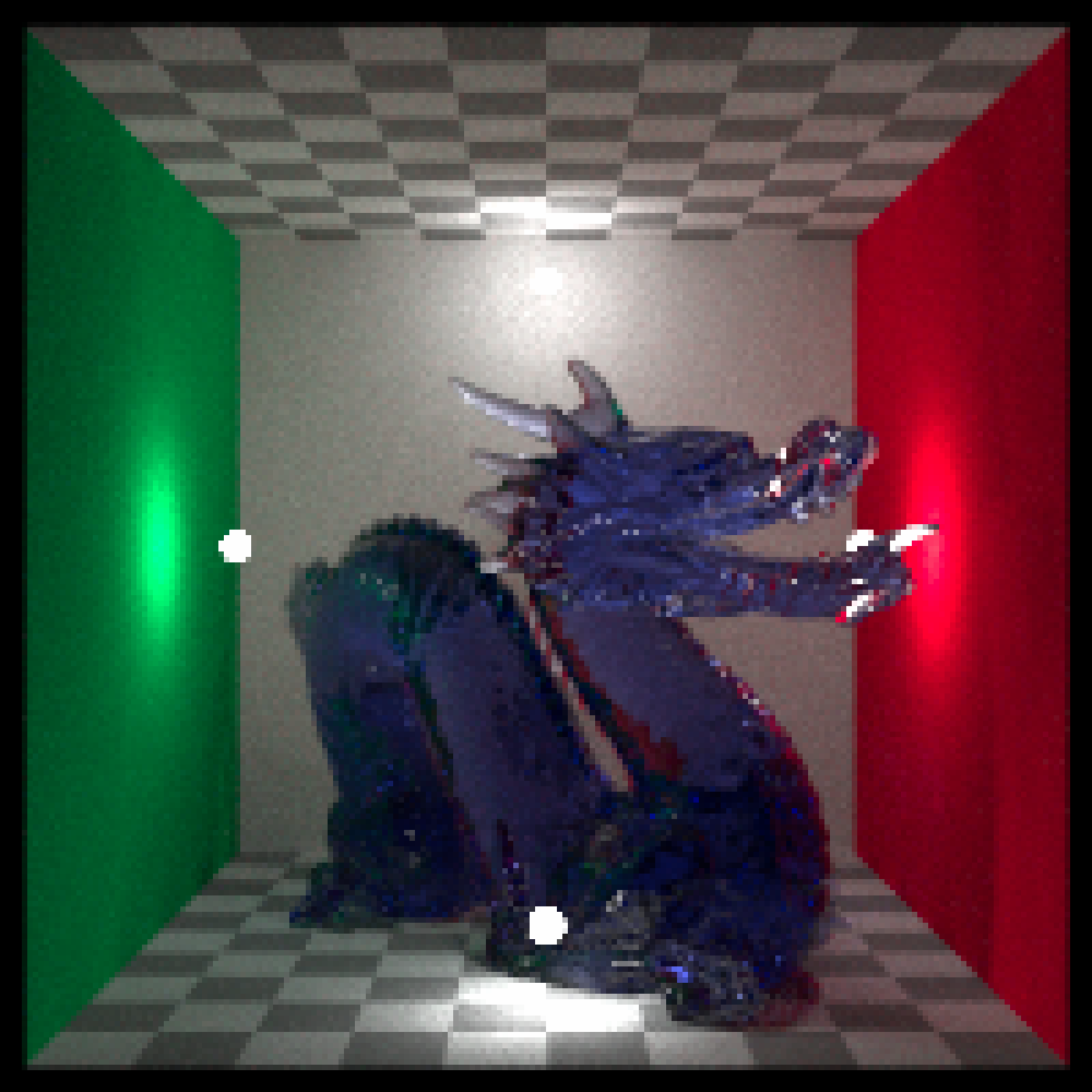#Perform a convolution with the default gaussian kernel
render_convolution(dragon, preview = TRUE)#Increase the width of the kernel
# \donttest{
render_convolution(dragon, kernel = 2, kernel_dim=21,kernel_extent=6, preview = TRUE)# }

#Perform edge detection using a edge detection kernel
# \donttest{
edge = matrix(c(-1,-1,-1,-1,8,-1,-1,-1,-1),3,3)
render_convolution(dragon, kernel = edge, preview = TRUE, absolute=FALSE)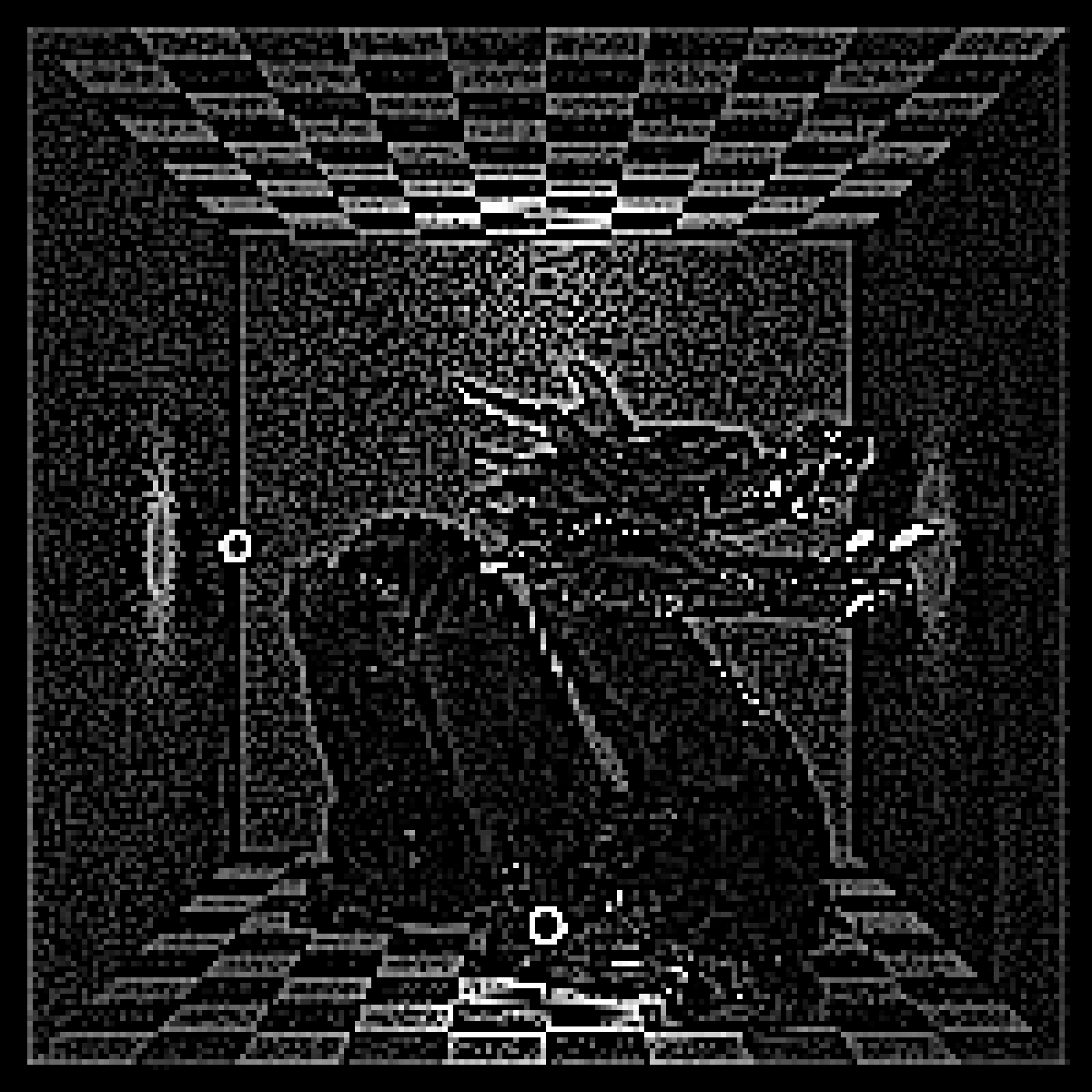# }

#Perform edge detection with Sobel matrices
# \donttest{
sobel1 = matrix(c(1,2,1,0,0,0,-1,-2,-1),3,3)
sobel2 = matrix(c(1,2,1,0,0,0,-1,-2,-1),3,3,byrow=TRUE)
sob1 = render_convolution(dragon, kernel = sobel1)
sob2 = render_convolution(dragon, kernel = sobel2)
sob_all = sob1 + sob2
plot_image(sob_all)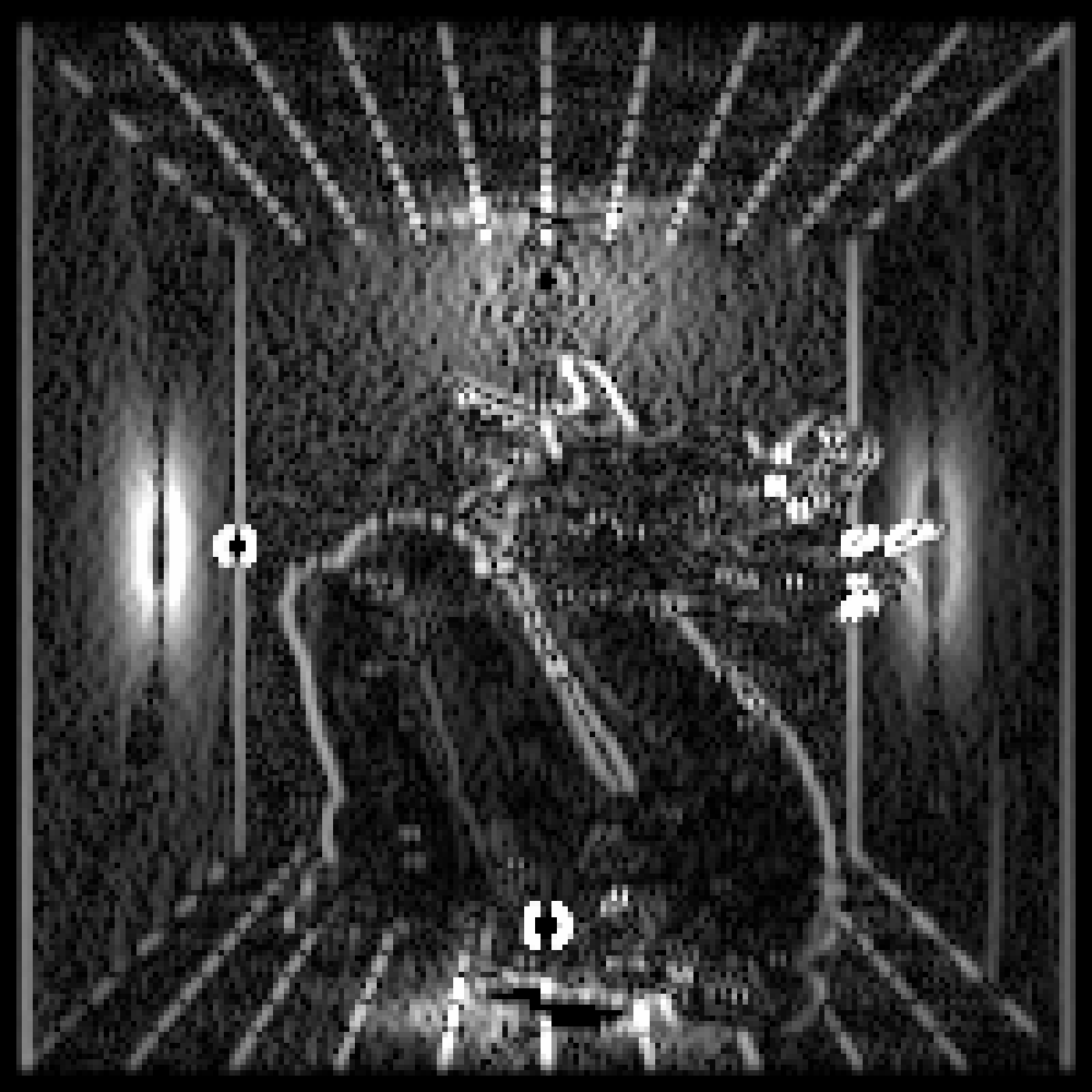# }
#Only perform the convolution on bright pixels (bloom)
# \donttest{
render_convolution(dragon, kernel = 5, kernel_dim=24, kernel_extent=24,
min_value=1, preview = TRUE)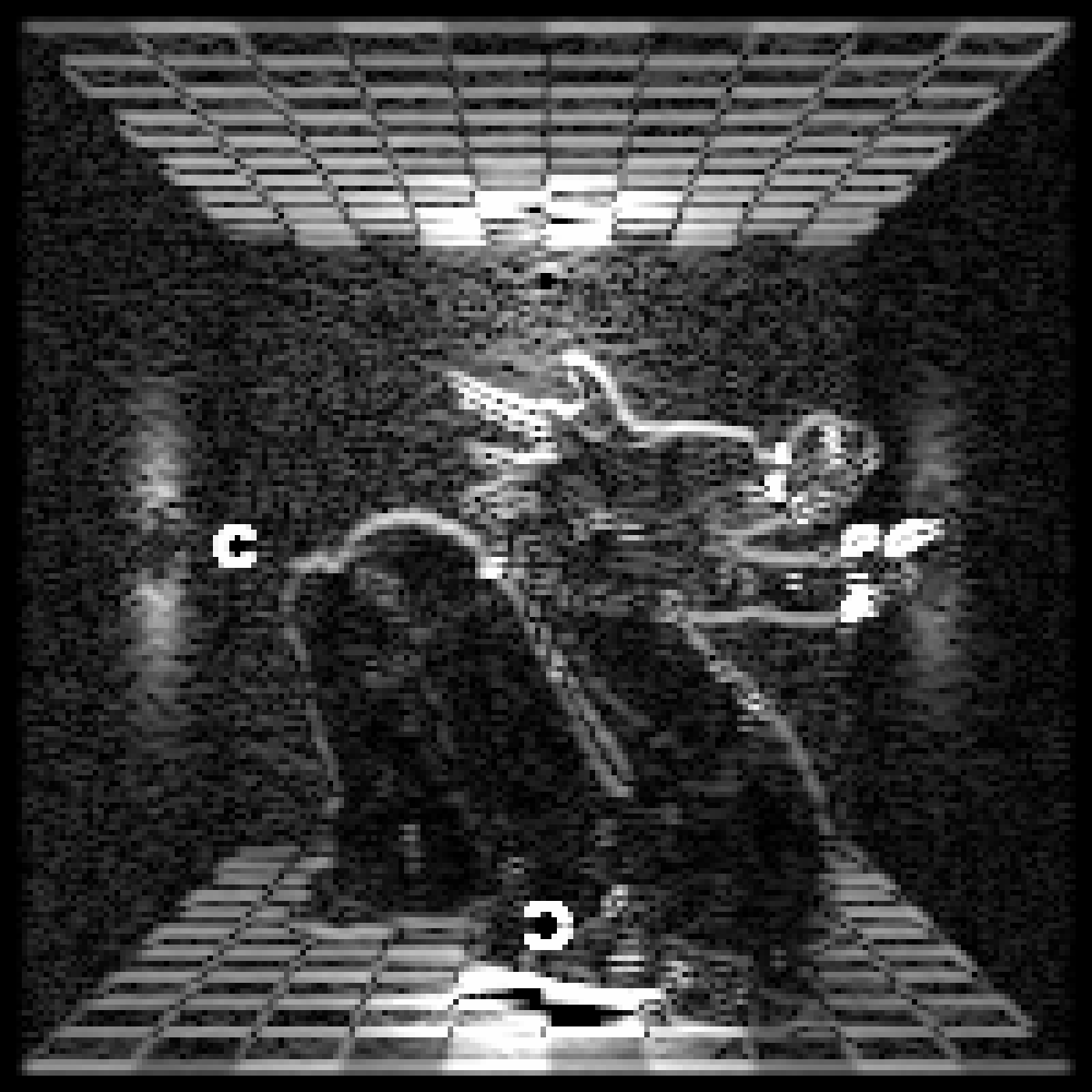# }

#Use a built-in kernel:
# \donttest{
render_convolution(dragon, kernel = generate_2d_exponential(falloff=2, dim=31, width=21),
preview = TRUE)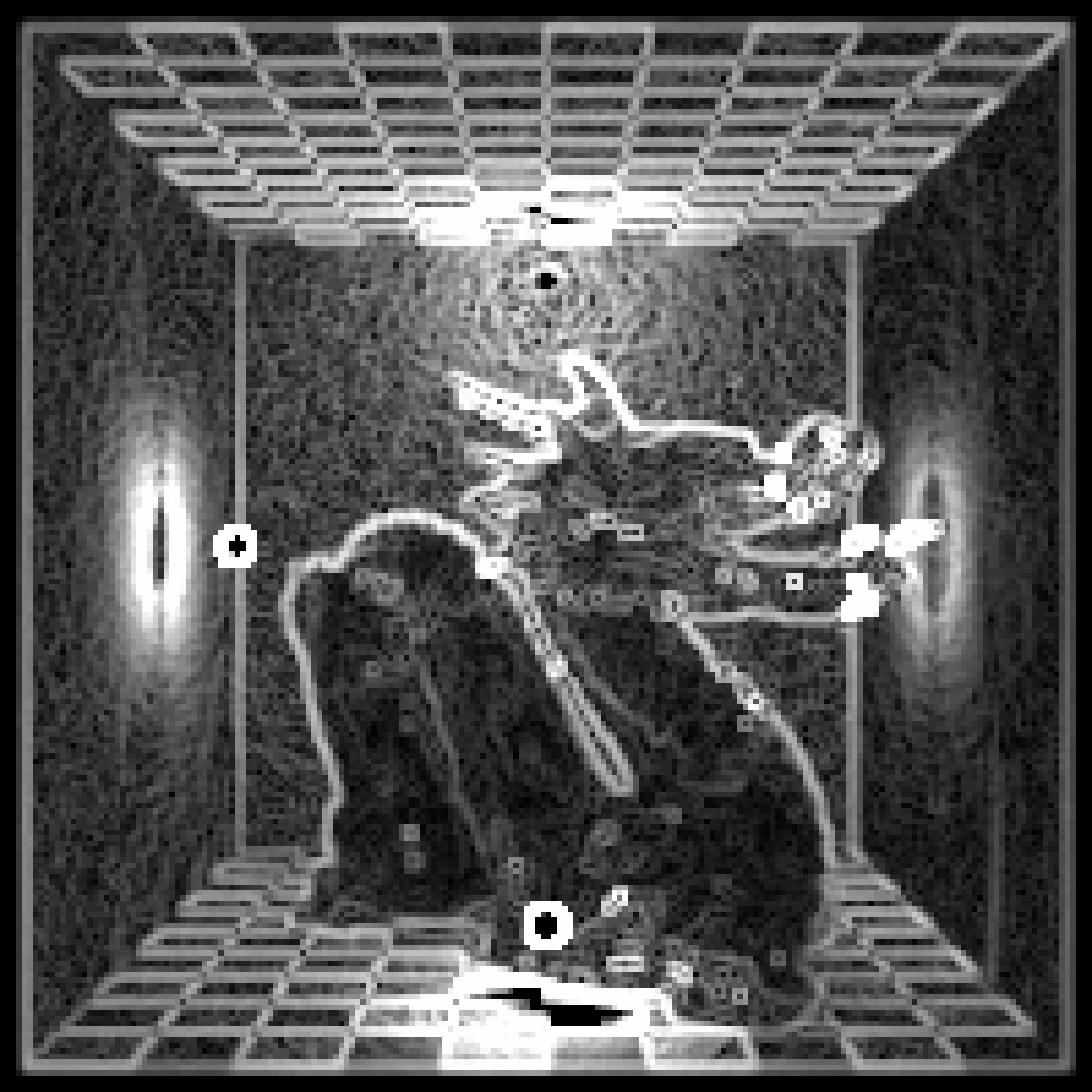# }

#We can also apply this function to matrices:
# \donttest{
volcano %>% image()volcano %>%
render_convolution(kernel=generate_2d_gaussian(sd=1,dim=31)) %>%
image()# }

#Use a custom kernel (in this case, an X shape):
custom = diag(10) + (diag(10)[,10:1])
# \donttest{
plot_image(custom)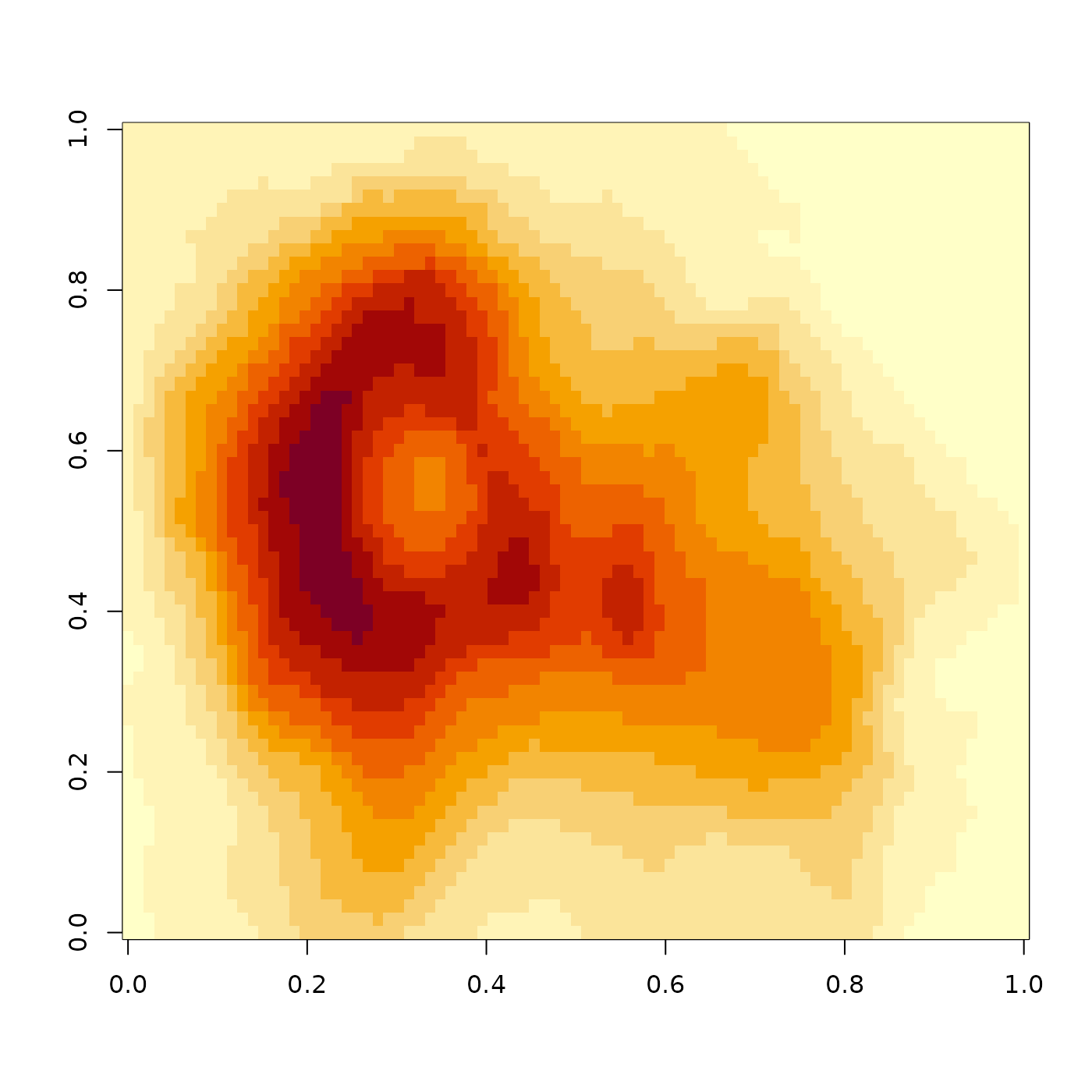render_convolution(dragon, kernel = custom, preview = TRUE)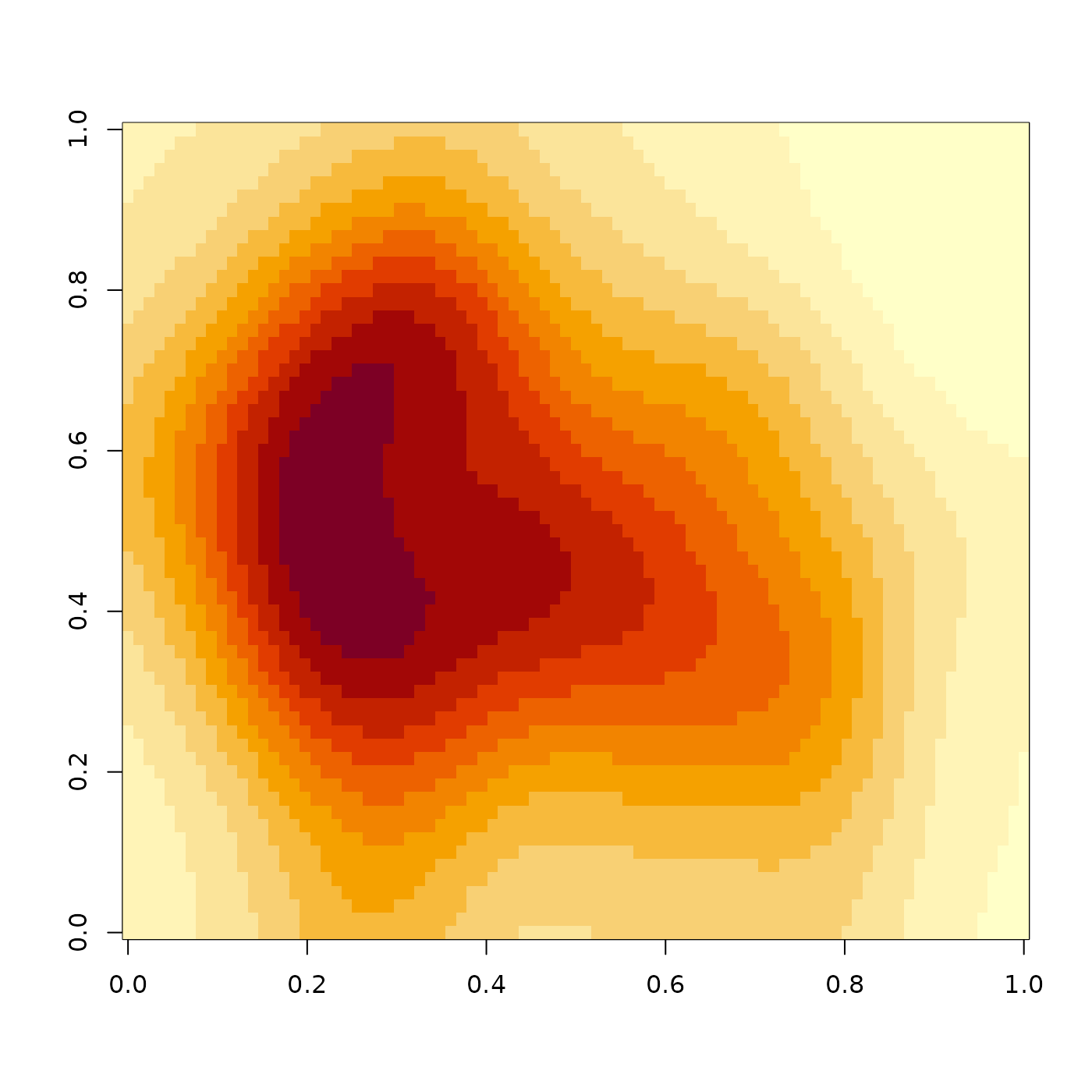# }
#end}